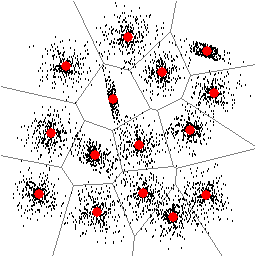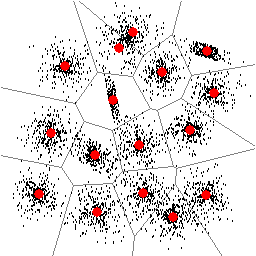# Algorithms in cluster analysis

Clustering is an important problem that must often be solved as a part of more complicated tasks in pattern recognition, image analysis and other fields of science and engineering. Clustering is also needed for designing a codebook in vector quantization. The clustering problem contains two sub problems:
• How many clusters there are in the data set
• Find the location of the clusters

## Clustering algorithms:

Clustering can be considered as NP-complete combinatoric optimization problem, for which optimal solutions can be found by branch-and-bound technique but in expontial time (Fränti and Virmajoki, ICPR 2002). Sub-optimal algorithms must therefore be used in practice. The existing methods can be roughly divided into hierarchical, iterative and optimization methods.

Iterative methods are usually based on the K-means algorithm (Forgy 1965), which applies two optimization steps iteratively: (i) calculate optimal partition for the given codebook, and (ii) calculate new codebook as the cluster centroids. There are many variants of the K-means including our faster implementation (Kaukoranta, Fränti and Nevalainen, IEEE-IP 2000). The fuzzy variant is known as fuzzy C-means (Dunn 1974), simulated annealing (Zeger and Gersho 1986), deterministic annealing (Rose et al 1990, Hoffmann and Buhmann 1997), among many others. These methods are based on nice and interesting theories, but it does not mean that they would work too well in practice (we have tried). Among those, the simulated annealing is still probably the one that works best in practice, whereas the plain K-means is still useful as it can be used as local fine-tuner in any other complicated algorithm (stay tuned and keep reading).

Hierarchical methods are either divisive or agglomerative. Divisive methods start by putting all data vectors in a sinlge cluster. New clusters are created by dividing existing ones. This approach involves two main design problems: which cluster to divide, and how the division is performed. The division can be made along a selected dimension of the vector space as in Median Cut algorithm (Heckbert, 1992) or along the principal axis (Wu and Zhang, 1991). We have studied the latter approach and proposed a method for locally fine-tuning the cluster boundaries are the divisions (Fränti and Kaukoranta, OE 1997). The divisive methods are usually fast, e.g. O(N log N), but more complicated than the agglomerative methods, for example.

Agglomerative clustering generate the clustering by a sequence of merge operations. The clustering starts by initializing each data vector as its own cluster. Two clusters are merged at each step and the process is repeated until the desired number of clusters is obtained. Ward's method (1963) selects the cluster pair to be merged so that it increases the given objective function value least. In the vector quantization context, this is known as the pairwise nearest neighbor (PNN) method due to Equitz (1989).

We have proposed a faster O(N^2) implementation with linear memory consumption of the PNN is by maintaining a pointer from each cluster to its nearest neighbor, and the corresponding merge cost value (Fränti, Kaukoranta and two Taiwanese, IEEE-IP 2000). Further speed-up can be achieved by using lazy update of the merge cost values (Kaukoranta, Fränti and Nevalainen, OE 1999), and by reducing the amount of work caused by the distance calculations (Virmajoki, Fränti and Kaukoranta, OE 2001).

The agglomerative is simple to implement and provides better clustering results than the divisive approach. It can also be combined with the Generalized Lloyd algorithm as proposed (de Garrido et al, 1995) or used as the merge phase in the split-and-merge algorithm (Kaukoranta, Fränti and Nevlainen, OE 1998) resulting in a good time-distortion performance. Recently, we have proposed an iterative shrinking method and shown that this method is conceptually more general than the merging appoach (Virmajoki, Fränti and Kaukoranta, ICIP 2002).

The best clustering results in term of minimizing the distortion function have been obtained by genetic algorithm with the agglomerative methods used in the crossover. The method was first reported by (Fränti et al, CompJ 1997) with effective but rather slow algorithm, and then simplified and made faster (Fränti, PRL 2000). The most recent results have been obtained by applying the iterative shrinking as the crossover with slight increase over the PNN method (Virmajoki et al, submitted). It is noted that the use of random crossover is not sufficient to compete the other methods.

Alternative approach has been offered by the Randomized Local Search (RLS) (Fränti and Kivijärvi, 2000). In terms of minimizing the distortion function it is almost as good as the Genetic Algorithms but the method is much simpler to implement. It used a simple trial-and-error approach, in which new candidate solutions are generated by random swapping of the code vectors, and then using K-means for fine-tuning the candidate solutions. Although this may sound slow and exhaustive approach, the new candidates can be generated fast and the number of iterations can be experimentally determined by moniotring the development of the distortion function. A good rule of thumb is to use as many iterations as there are data vectors - about 1000 iterations for data sets of size 1000-5000 has found to be more than enough.

The RLS method introduced has also been succesfully applied for the classification of bacteria (Fränti et al, Biosystems 2000). To sum up, it is the algorithm we recommend for practitioners. At least we use it as the basic algorithm when we need to cluster data.

## Determining the number of clusters:

Determination of the number of clusters in data requires that we have both an algorithm that can seek for the correct number and a criterion that is capable of recognizing the correct number of clusters. The simplest algorithm is to use an existing algortihm for fixed number of clusters in a loop and select the best solution with some criterium. This brute force search is quaranteed to work but is also the slowest method.

Stepwise clustering algorithm for reducing the work load required by the Brute Force has been proposed (Kärkkäinen and Fränti, ACIVS 2002). The idea is to utilize the previous solution as a starting point when solving the next clustering problem with different number of clusters. A stopping criterion is applied to estimate the potential improvement of the algorithm, and to stop the iteration when the estimated further improvement stays below a predefined threshold value.

There are also two methods that solves the number and location of the clusters jointly. We know two of them that we dare to mention. The Competitive agglomeration (Frigui and Krishnapuram, 1997) decreases the number of clusters until there are no clusters smaller than a predefined threshold value. The drawback is that the threshold value must be experimentally determined. Our approach is the Dynamic Local Search (Kärkkäinen and Fränti, ICPR 2002) that solves the number and location of the clusters jointly. The algorithm uses a set of basic operations, such as cluster addition, removal and swapping. The rest is the same trial-and-error approach as in the RLS.

The more difficult question is the choice of the evaluation function that is to be minimized. For a given application, the criterion can be based on the principle of Minimum Description Length (MDL). Otherwise, the criterion must be based on certain assumption of data normalization and spherical clusters. Basically the function should correlate with high inter cluster distance, and low intra cluster diversity. In the case of Binary data, we have applied Stochastic Complexity (Gyllenberg et al, 1997). We have proposed Delta-SC distance based on the properties of the measure for better clustering performance (Fränti, Xu and Kärkkäinen, PRL 2003).

### Sources:Figure 1: Found locations of 15 clusters. Figure 2: Found locations of 16 clusters.